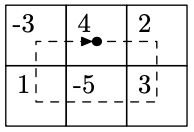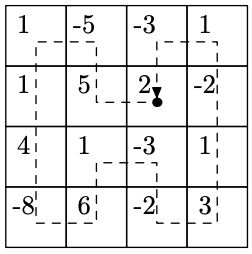시간 제한 메모리 제한 제출 정답 맞은 사람 정답 비율
2 초 512 MB 2 1 1 50.000%

## 문제

Byteland is a rectangle of $n\times m$, divided into $n\cdot m$ square provinces. Recently in Byteland tax reform was carried out, as a result of which the number $a[i, j]$ was fixed for each province. If $a[i, j] > 0$, then the province in the square $(i, j)$ must pay $a[i, j]$ bytecoins every month to the budget. If $a[i, j] < 0$, then the province $(i, j)$ is subsidized and receives $-a[i, j]$ bytecoins from the budget.

To collect taxes, the government has developed the following scheme. In one of the provinces a treasury will be built. Every month a tax collector will leave this building. He will go round all the provinces, collecting taxes and giving out subsidies, and then going back to the treasury. His path must satisfy the following properties:

• the path must begin in the province in which the treasury is located,
• the path must end in a province that has a common side with the province in which the treasury is located,
• each province should be visited exactly once,
• neighboring provinces along the path should have a common side.

The government wants to choose a province for the treasury and a path for the collector in such a way that for each subsidized province the collector can give them the right amount of money from the previously collected. Help them build such a path or say that it is impossible.

## 입력

The first line contains two integers $n$ and $m$ --- the size of Byteland ($2 \le n, m \le 300$).

Each of next $n$ rows consists of $m$ integers $a[i, j]$. These lines describe the provinces: $a[i, j]$ is the value by which the number of bytecoins owned by the collector will change when visiting the province located at $(i, j)$ ($1 \le |a[i, j]| \le 10\,000$).

## 출력

If solution exists, output $n \cdot m$ pairs of numbers, the coordinates of the provinces that the collector should visit in the order in which he should visit them. If there is no solution, output $-1$ .

## 서브태스크

번호 배점 제한
1 23

$n = 2$, $m \le 300$

2 15

$n \le 300$, $m \le 300$, all $a_{i, j} > 0$

3 29

$n \le 300$, $m \le 300$, exactly one $a_{i, j} < 0$

4 33

$n \le 300$, $m \le 300$

## 예제 입력 1

2 3
-3 4 2
1 -5 3


## 예제 출력 1

1 2
1 3
2 3
2 2
2 1
1 1


## 예제 입력 2

4 4
1 -5 -3 1
1 5 2 -2
4 1 -3 1
-8 6 -2 3


## 예제 출력 2

2 3
2 2
1 2
1 1
2 1
3 1
4 1
4 2
3 2
3 3
4 3
4 4
3 4
2 4
1 4
1 3


## 예제 입력 3

2 2
1 -2
-1 1


## 예제 출력 3

-1


## 예제 입력 4

3 3
1 1 1
1 1 1
1 1 1


## 예제 출력 4

-1


## 힌트

Path for the first example:Sum of bytecoins that collector has after each province: 4, 6, 9, 4, 5, 2.

Path for the second example:Sum of bytecoins that collector has after each province: 2, 7, 2, 3, 4, 8, 0, 6, 7, 4, 2, 5, 6, 4, 5, 2.

## 채점 및 기타 정보

• 예제는 채점하지 않는다.